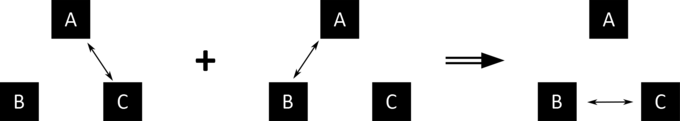## The Zeroth Law of Thermodynamics

The Zeroth Law of Thermodynamics states that systems in thermal equilibrium are at the same temperature.

### Learning Objectives

Identify major implications of the Zeroth Law of Thermodynamics

### Key Takeaways

#### Key Points

• Assuming A, B, and C are three systems, if A and C are in thermal equilibrium, and A and B are in thermal equilibrium, then B and C are in thermal equilibrium.
• Two systems are in thermal equilibrium if they could transfer heat between each other, but do not.
• The Zeroth Law of Thermodynamics implies that temperature is a quantity worth measuring.

#### Key Terms

• thermal equilibrium: Two systems are in thermal equilibrium if they could transfer heat between each other, but don’t.
• zeroth law of thermodynamics: Let A, B and C be three systems. If A and C are in thermal equilibrium, and A and B are in thermal equilibrium, then B and C are in thermal equilibrium.

### The Zeroth Law of Thermodynamics

There are a few ways to state the Zeroth Law of Thermodynamics, but the simplest is as follows: systems that are in thermal equilibrium exist at the same temperature.

Systems are in thermal equilibrium if they do not transfer heat, even though they are in a position to do so, based on other factors. For example, food that’s been in the refrigerator overnight is in thermal equilibrium with the air in the refrigerator: heat no longer flows from one source (the food) to the other source (the air) or back.

What the Zeroth Law of Thermodynamics means is that temperature is something worth measuring, because it indicates whether heat will move between objects. This will be true regardless of how the objects interact. Even if two objects don’t touch, heat may still flow between them, such as by radiation (as from a heat lamp). However, according to the Zeroth Law of Thermodynamics, if the systems are in thermal equilibrium, no heat flow will take place.

There are more formal ways to state the Zeroth Law of Thermodynamics, which is commonly stated in the following manner:

Let A, B, and C be three systems. If A and C are in thermal equilibrium, and A and B are in thermal equilibrium, then B and C are in thermal equilibrium.

This statement is represented symbolically in. Temperature is not mentioned explicitly, but it’s implied that temperature exists. Temperature is the quantity that is always the same for all systems in thermal equilibrium with one another.Zeroth Law of Thermodynamics: The double arrow represents thermal equilibrium between systems. If systems A and C are in equilibrium, and systems A and B are in equilibrium, then systems B and C are in equilibrium. The systems A, B, and C are at the same temperature.